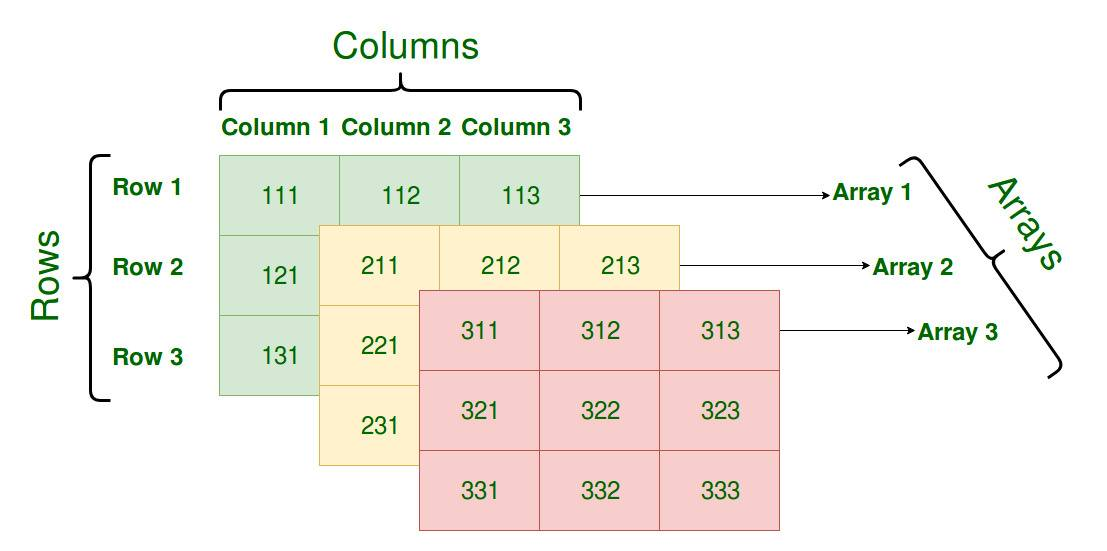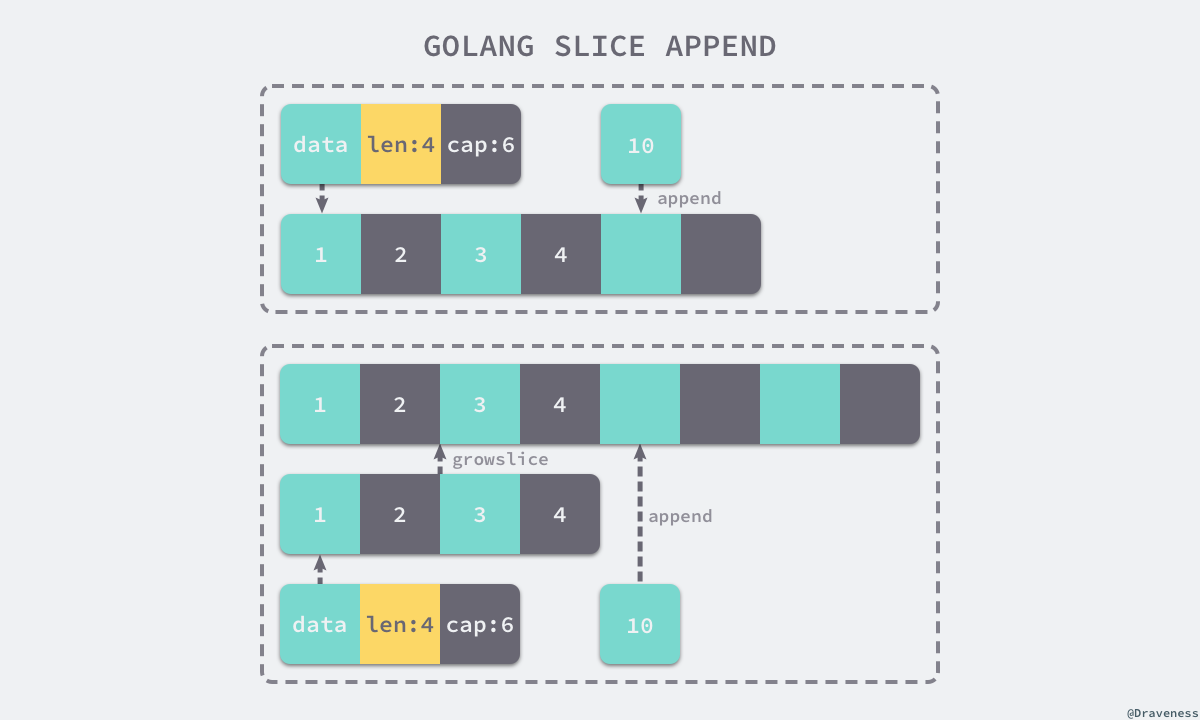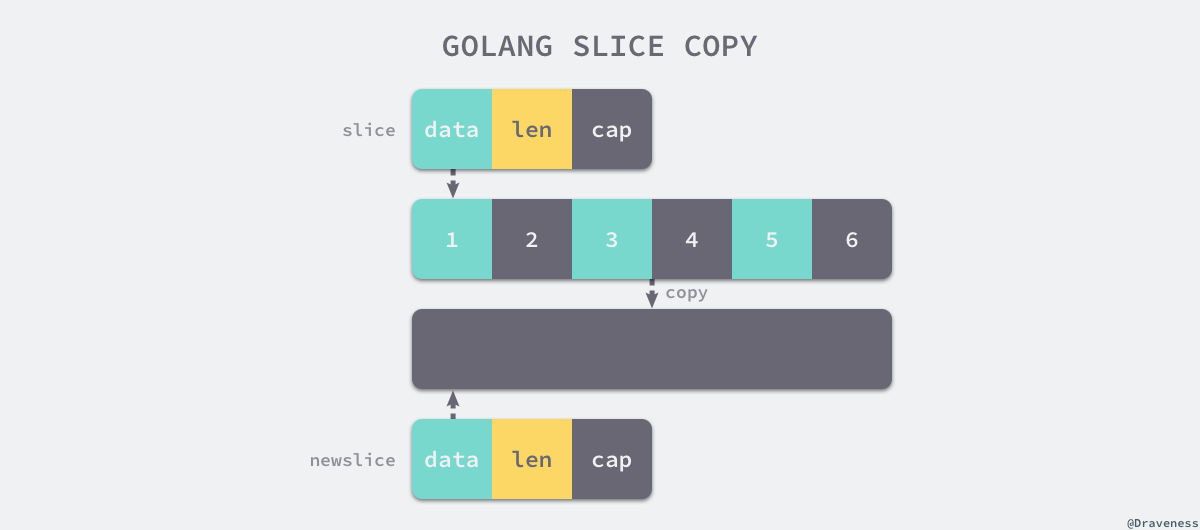# 3.1 数组和切片

## 1. 数组``````int
interface{}
``````

Go 语言中数组的大小在初始化之后就无法改变，数组存储元素的类型相同，但是大小不同的数组类型在 Go 语言看来也是完全不同的，只有两个条件都相同才是同一个类型。

``````func NewArray(elem *Type, bound int64) *Type {
if bound < 0 {
Fatalf("NewArray: invalid bound %v", bound)
}
t := New(TARRAY)
t.Extra = &Array{Elem: elem, Bound: bound}
t.SetNotInHeap(elem.NotInHeap())
return t
}
``````

### 1.1. 创建

Go 语言中的数组有两种不同的创建方式，一种是我们显式指定数组的大小，另一种是编译器通过源代码自行推断数组的大小：

``````arr1 := int{1, 2, 3}
arr2 := [...]int{1, 2, 3}
``````

#### 上限推导

``````func typecheckcomplit(n *Node) (res *Node) {
// ...

switch t.Etype {
case TARRAY, TSLICE:
var length, i int64
nl := n.List.Slice()
for i2, l := range nl {
i++
if i > length {
length = i
}
}

if t.IsDDDArray() {
t.SetNumElem(length)
}
}
}
``````

#### 语句转换

``````func anylit(n *Node, var_ *Node, init *Nodes) {
t := n.Type
switch n.Op {
case OSTRUCTLIT, OARRAYLIT:
if n.List.Len() > 4 {
vstat := staticname(t)

fixedlit(inNonInitFunction, initKindStatic, n, vstat, init)

a := nod(OAS, var_, vstat)
a = typecheck(a, ctxStmt)
a = walkexpr(a, init)
init.Append(a)
break
}

fixedlit(inInitFunction, initKindLocalCode, n, var_, init)
// ...
}
}
``````
1. 当元素数量小于或者等于 4 个时，会直接将数组中的元素放置在栈上；
2. 当元素数量大于 4 个时，会将数组中的元素放置到静态区并在运行时取出；

``````func fixedlit(ctxt initContext, kind initKind, n *Node, var_ *Node, init *Nodes) {
var splitnode func(*Node) (a *Node, value *Node)
// ...

for _, r := range n.List.Slice() {
a, value := splitnode(r)

a = nod(OAS, a, value)
a = typecheck(a, ctxStmt)
switch kind {
case initKindStatic:
genAsStatic(a)
case initKindLocalCode:
a = orderStmtInPlace(a, map[string][]*Node{})
a = walkstmt(a)
init.Append(a)
default:
}
}
}
``````

``````var arr int
arr = 1
arr = 2
arr = 3
``````

``````func fixedlit(ctxt initContext, kind initKind, n *Node, var_ *Node, init *Nodes) {
var splitnode func(*Node) (a *Node, value *Node)
// ...

for _, r := range n.List.Slice() {
a, value := splitnode(r)

setlineno(value)
a = nod(OAS, a, value)
a = typecheck(a, ctxStmt)
switch kind {
case initKindStatic:
genAsStatic(a)
default:
}

}
}
``````

``````var arr int
statictmp_0 = 1
statictmp_0 = 2
statictmp_0 = 3
statictmp_0 = 4
statictmp_0 = 5
arr = statictmp_0
``````

### 1.2. 访问和赋值``````func typecheck1(n *Node, top int) (res *Node) {
switch n.Op {
case OINDEX:
ok |= ctxExpr
l := n.Left
r := n.Right
t := l.Type
switch t.Etype {
case TSTRING, TARRAY, TSLICE:
why := "string"
if t.IsArray() {
why = "array"
} else if t.IsSlice() {
why = "slice"
}

if n.Right.Type != nil && !n.Right.Type.IsInteger() {
yyerror("non-integer %s index %v", why, n.Right)
break
}

if !n.Bounded() && Isconst(n.Right, CTINT) {
x := n.Right.Int64()
if x < 0 {
yyerror("invalid %s index %v (index must be non-negative)", why, n.Right)
} else if t.IsArray() && x >= t.NumElem() {
yyerror("invalid array index %v (out of bounds for %d-element array)", n.Right, t.NumElem())
} else if Isconst(n.Left, CTSTR) && x >= int64(len(n.Left.Val().U.(string))) {
yyerror("invalid string index %v (out of bounds for %d-byte string)", n.Right, len(n.Left.Val().U.(string)))
}
}
}
//...
}
}
``````

``````PtrIndex <t> ptr idx
``````

``````LocalAddr {sym} base _
PtrIndex <t> ptr idx
Store {t} ptr val mem
``````

## 2. 切片

``````[]int
[]interface{}
``````

``````func NewSlice(elem *Type) *Type {
if t := elem.Cache.slice; t != nil {
if t.Elem() != elem {
Fatalf("elem mismatch")
}
return t
}

t := New(TSLICE)
t.Extra = Slice{Elem: elem}
elem.Cache.slice = t
return t
}
``````

### 2.1. 结构

``````type SliceHeader struct {
Data uintptr
Len  int
Cap  int
}
``````

`Data` 作为一个指针指向的数组其实就是一片连续的内存空间，这片内存空间可以用于存储切片中保存的全部元素，数组其实就是一片连续的内存空间，数组中的元素只是逻辑上的概念，底层存储其实都是连续的，所以我们可以将切片理解成一片连续的内存空间加上长度与容量标识。### 2.2. 初始化

``````slice := []int{1, 2, 3}
slice := make([]int, 10)
``````

#### 字面量

``````var vstat int
vstat = 1
vstat = 2
vstat = 3
var vauto *int = new(int)
*vauto = vstat
slice := vauto[:]
``````
1. 根据切片中的元素数量对底层数组的大小进行推断并创建一个数组；
2. 将这些字面量元素存储到初始化的数组中；
3. 创建一个同样指向 `int` 类型的数组指针；
4. 将静态存储区的数组 `vstat` 赋值给 `vauto` 指针所在的地址；
5. 通过 `[:]` 操作获取一个底层使用 `vauto` 的切片；

`[:]` 以及类似的操作 `[:10]` 其实都会在 SSA 代码生成 阶段被转换成 `OpSliceMake` 操作，这个操作会接受四个参数创建一个新的切片，切片元素类型、数组指针、切片大小和容量。

#### 关键字

``````func typecheck1(n *Node, top int) (res *Node) {
switch n.Op {
// ...
case OMAKE:
args := n.List.Slice()

i := 1
switch t.Etype {
case TSLICE:
if i >= len(args) {
yyerror("missing len argument to make(%v)", t)
return n
}

l = args[i]
i++
var r *Node
if i < len(args) {
r = args[i]
}

// ...
if Isconst(l, CTINT) && r != nil && Isconst(r, CTINT) && l.Val().U.(*Mpint).Cmp(r.Val().U.(*Mpint)) > 0 {
yyerror("len larger than cap in make(%v)", t)
return n
}

n.Left = l
n.Right = r
n.Op = OMAKESLICE
}
// ...
}
}
``````

`make` 参数的检查都是在 `typecheck1` 函数中完成的，它不仅会检查 `len`，而且会保证传入的容量 `cap` 一定大于或者等于 `len`；随后的中间代码生成阶段会把这里的 `OMAKESLICE` 类型的操作都转换成如下所示的函数调用：

``````makeslice(type, len, cap)
``````

``````var arr int
n := arr[:3]
``````

``````func makeslice(et *_type, len, cap int) unsafe.Pointer {
mem, overflow := math.MulUintptr(et.size, uintptr(cap))
if overflow || mem > maxAlloc || len < 0 || len > cap {
mem, overflow := math.MulUintptr(et.size, uintptr(len))
if overflow || mem > maxAlloc || len < 0 {
panicmakeslicelen()
}
panicmakeslicecap()
}

return mallocgc(mem, et, true)
}
``````

``````func typecheck1(n *Node, top int) (res *Node) {
switch n.Op {
// ...
switch
t := n.Type
n.Left = typecheck(n.Left, ctxExpr)
l := typecheck(n.List.First(), ctxExpr)
c := typecheck(n.List.Second(), ctxExpr)
l = defaultlit(l, types.Types[TINT])
c = defaultlit(c, types.Types[TINT])

n.List.SetFirst(l)
n.List.SetSecond(c)
// ...
}
}
``````

`OSLICEHEADER` 操作会创建一个如下所示的结构体，其中包含数组指针、切片长度和容量，它是切片在运行时的表示：

``````type SliceHeader struct {
Data uintptr
Len  int
Cap  int
}
``````

### 2.3. 访问

``````func (s *state) expr(n *Node) *ssa.Value {
switch n.Op {
case OLEN, OCAP:
switch {
case n.Left.Type.IsSlice():
op := ssa.OpSliceLen
if n.Op == OCAP {
op = ssa.OpSliceCap
}
return s.newValue1(op, types.Types[TINT], s.expr(n.Left))
// ...
}
// ...
}
}
``````

``````func (s *state) expr(n *Node) *ssa.Value {
switch n.Op {
case OINDEX:
switch {
case n.Left.Type.IsSlice():
// ...
}
// ...
}
}
``````

### 2.4. 追加

``````ptr, len, cap := slice
newlen := len + 3
if newlen > cap {
ptr, len, cap = growslice(slice, newlen)
newlen = len + 3
}
*(ptr+len) = 1
*(ptr+len+1) = 2
*(ptr+len+2) = 3
return makeslice(ptr, newlen, cap)
``````

``````a := &slice
ptr, len, cap := slice
newlen := len + 3
if uint(newlen) > uint(cap) {
newptr, len, newcap = growslice(slice, newlen)
vardef(a)
*a.cap = newcap
*a.ptr = newptr
}
newlen = len + 3
*a.len = newlen
*(ptr+len) = 1
*(ptr+len+1) = 2
*(ptr+len+2) = 3
````````````func growslice(et *_type, old slice, cap int) slice {
newcap := old.cap
doublecap := newcap + newcap
if cap > doublecap {
newcap = cap
} else {
if old.len < 1024 {
newcap = doublecap
} else {
for 0 < newcap && newcap < cap {
newcap += newcap / 4
}
if newcap <= 0 {
newcap = cap
}
}
}
``````

1. 如果期望容量大于当前容量的两倍就会使用期望容量；
2. 如果当前切片容量小于 1024 就会将容量翻倍；
3. 如果当前切片容量大于 1024 就会每次增加 25% 的容量，直到新容量大于期望容量；

``````    var overflow bool
var lenmem, newlenmem, capmem uintptr
switch {
// ...
default:
lenmem = uintptr(old.len) * et.size
newlenmem = uintptr(cap) * et.size
capmem, overflow = math.MulUintptr(et.size, uintptr(newcap))
capmem = roundupsize(capmem)
newcap = int(capmem / et.size)
}

var p unsafe.Pointer
if et.kind&kindNoPointers != 0 {
p = mallocgc(capmem, nil, false)
} else {
p = mallocgc(capmem, et, true)
if writeBarrier.enabled {
bulkBarrierPreWriteSrcOnly(uintptr(p), uintptr(old.array), lenmem)
}
}
memmove(p, old.array, lenmem)

return slice{p, old.len, newcap}
}
``````

``````TEXT runtime·memclrNoHeapPointers(SB), NOSPLIT, \$0-16
MOVD ptr+0(FP), R0
MOVD n+8(FP), R1

loop:
Loop
Get R1
I64Eqz
If
RET
End

Get R0
I32WrapI64
I64Const \$0
I64Store8 \$0

Get R0
I64Const \$1
Set R0

Get R1
I64Const \$1
I64Sub
Set R1

Br loop
End
UNDEF
``````

`growslice` 函数最终会返回一个新的 `slice` 结构，其中包含了新的数组指针、大小和容量，这个返回的三元组最终会改变原有的切片，帮助 `append` 完成元素追加的功能。

### 2.5. 拷贝

``````func slicecopy(to, fm slice, width uintptr) int {
if fm.len == 0 || to.len == 0 {
return 0
}

n := fm.len
if to.len < n {
n = to.len
}

if width == 0 {
return n
}

// ...

size := uintptr(n) * width
if size == 1 {
*(*byte)(to.array) = *(*byte)(fm.array)
} else {
memmove(to.array, fm.array, size)
}
return n
}
``````## 5. 其他

### 5.2. 微信公众号